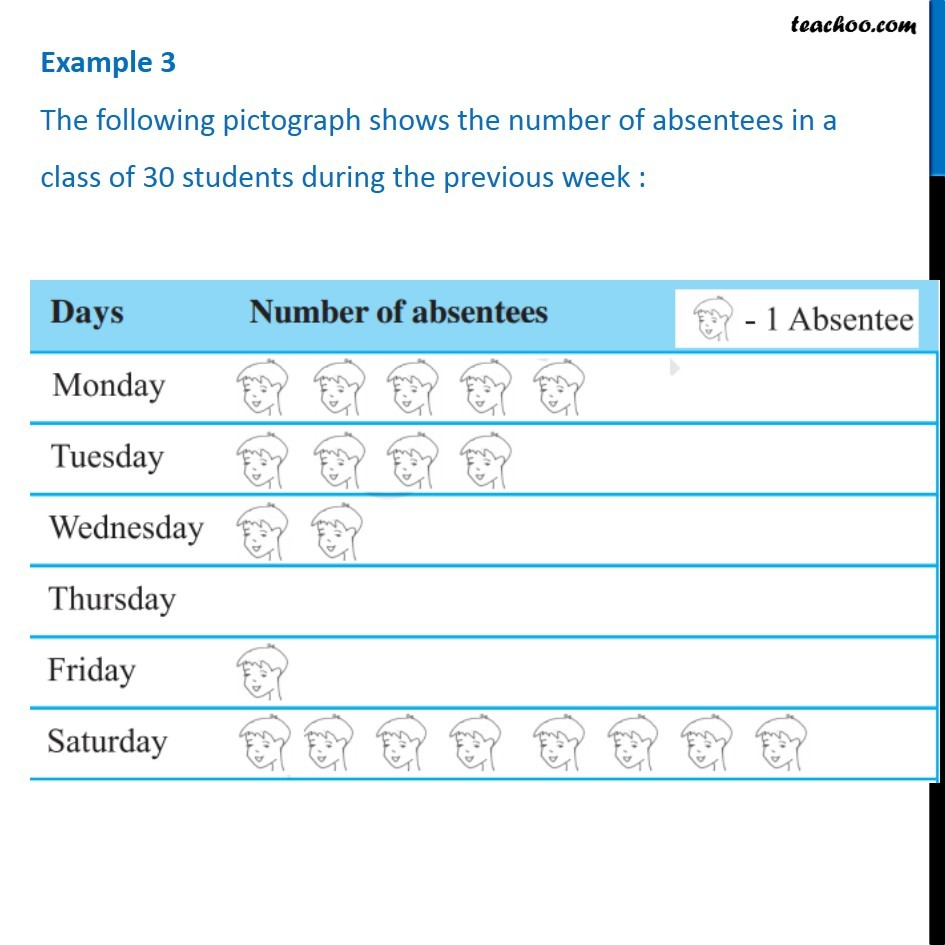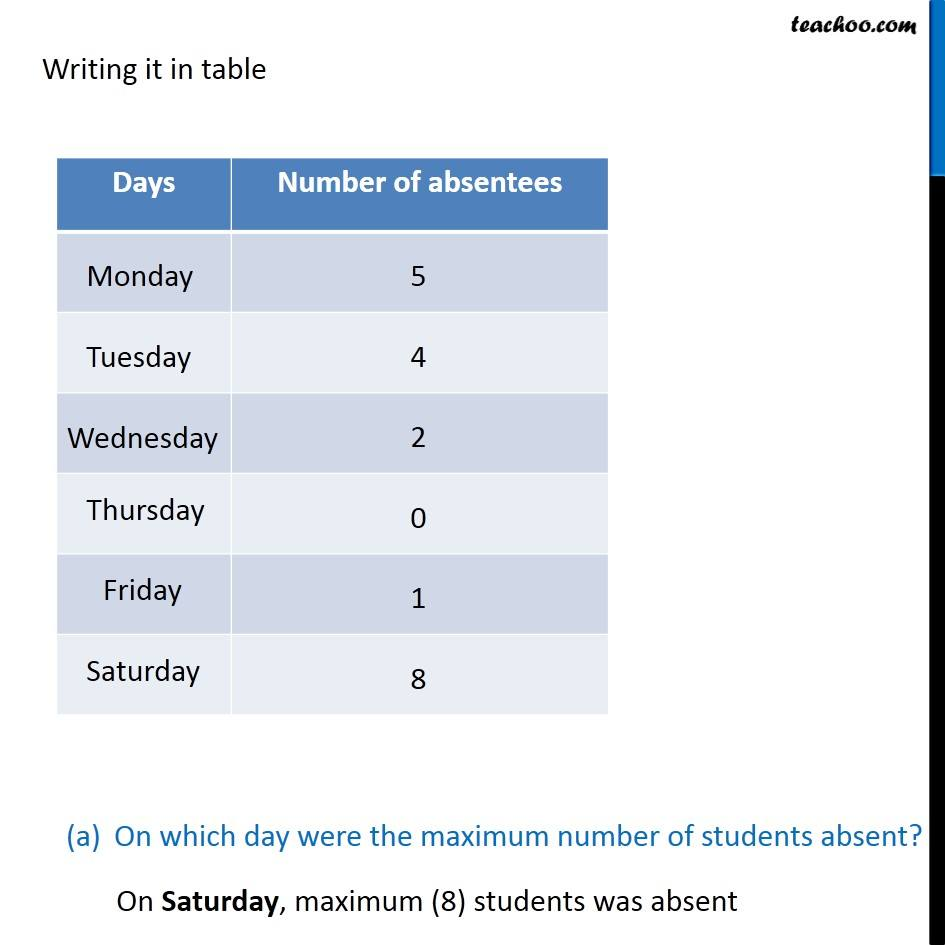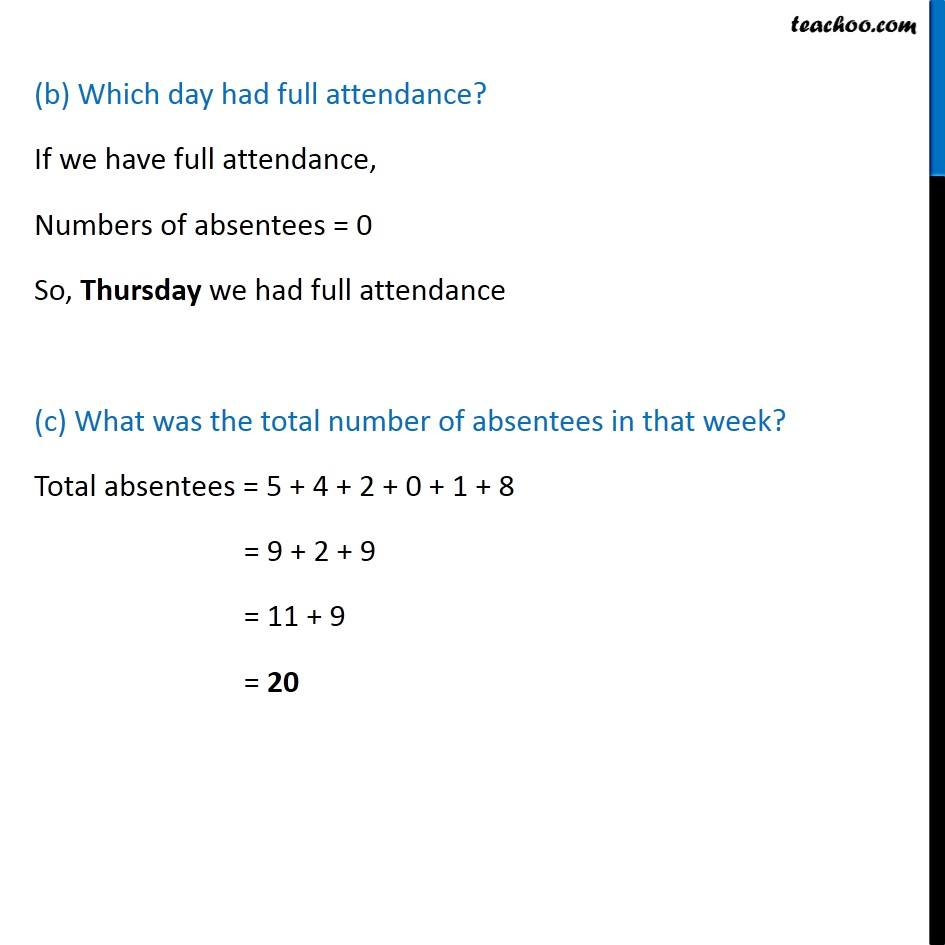Examples

Chapter 9 Class 6 Data Handling
Serial order wiseLearn in your speed, with individual attention - Teachoo Maths 1-on-1 Class

### Transcript

Example 3 The following pictograph shows the number of absentees in a class of 30 students during the previous week : Writing it in table On which day were the maximum number of students absent? On Saturday, maximum (8) students was absent (b) Which day had full attendance? If we have full attendance, Numbers of absentees = 0 So, Thursday we had full attendance (c) What was the total number of absentees in that week? Total absentees = 5 + 4 + 2 + 0 + 1 + 8 = 9 + 2 + 9 = 11 + 9 = 20